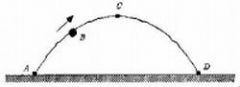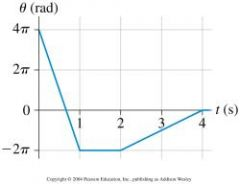• Shuffle
Toggle On
Toggle Off
• Alphabetize
Toggle On
Toggle Off
• Front First
Toggle On
Toggle Off
• Both Sides
Toggle On
Toggle Off
Toggle On
Toggle Off
Front

## Card Range To Study

throughPlay buttonPlay buttonProgress

1/24

Click to flip

Use LEFT and RIGHT arrow keys to navigate between flashcards;

Use UP and DOWN arrow keys to flip the card;

H to show hint;

 A 3 kg ball is thrown horizontally with a speed of 20 m/s over a level field. At the same time, a 1 kg ball of the same size is thrown horizontally at 10 m/s. Which ball hits the ground first? They both hit at the same time A baseball is hit upward and travels along a parabolic arc before it strikes the ground. Which one of the following statements is necessarily true? The x-component of the velocity of the ball is the same throughout the ball's flight. The x components are the same; the y component at C is zero.How do the x and y components of the velocity vector of the ball compare at the points B and C? The ball's velocity is perpendicular to its acceleration.Which statement is true concerning the ball when it is at C, the highest point in its trajectory? The velocity components have the same magnitudes at both points, but the directions of the y components are reversed.How do the x and y components of the velocity vector of the ball compare at the points A and D? A bicyclist is riding at a constant speed along a straight line path. The rider throws a ball straight up to a height a few meters above her head. Ignoring air resistance, where will the ball land? in the same hand that threw the ball The statement that “Newton’s laws of motion are valid in all inertial reference frames” is the Galilean principle of relativity A passenger at rest on a moving subway train fires a bullet straight up from his seat. The event is viewed by observers at rest on the station platform as the train moves by the platform with constant velocity. What is the trajectory of the bullet as described by the observers on the platform? a parabolic path A car travels at a constant speed around a circular track. Which of the following statements are true? (more than one may be correct) The velocity of the car is changing. 6pi rad/secThe figure shows the angular position vs time graph for a body undergoing circular motion. What is the maximum angular speed between 0-4 seconds? A race car is traveling at constant speed around a circular track. What happens to the centripetal acceleration of the car if the speed is doubled? It increases by a factor of 4. Which of the following statements are correct regarding the motion of an object in two dimensions? There may be more than one. Tangential acceleration is nonzero only if the object changes speed. Radial acceleration is zero only if the object moves in a straight line. A satellite is in a circular orbit about the earth at an altitude at which air resistance is negligible. Which one of the following statements is true? Neglect the influence of other planets and the Moon. There are two forces acting on the satellite, and their resultant is not zero. Which one of the following does not effect the maximum speed at which a car can go around a curve without skidding? The mass of the car. A ball on the end of a string moves in a vertical circle such that the force on the ball from the tension in the string is never zero. Which one of the following statements regarding the directions of the force of tension and the force of gravity on the ball is correct? At the top they are in the same direction, and at the bottom they are in the opposite direction. Two artificial satellites have the same orbital radius around a distant planet, but satellite A has twice the mass of satellite B. Which one of the following statements is correct? Satellite A has the same orbital period of satellite B. A satellite orbiting the moon very near the surface has a period of 110 minutes. What is the acceleration due to gravity (g) on the moon? Note: r(moon) = 1,738,000 m. 1.58 m/s^2 Which one of the following does not effect the maximum speed at which a car can go around a curve without skidding? The mass of the car. A satellite is in a circular orbit about the earth at an altitude at which air resistance is negligible. Which one of the following statements is true? Neglect the influence of other planets and the Moon. There are two forces acting on the satellite, and their resultant is not zero. A ball on the end of a string moves in a vertical circle such that the force on the ball from the tension in the string is never zero. Which one of the following statements regarding the directions of the force of tension and the force of gravity on the ball is correct? At the top they are in the same direction, and at the bottom they are in the opposite direction. Two artificial satellites have the same orbital radius around a distant planet, but satellite A has twice the mass of satellite B. Which one of the following statements is correct? Satellite A has the same orbital period of satellite B. A satellite orbiting the moon very near the surface has a period of 110 minutes. What is the acceleration due to gravity (g) on the moon? Note: r(moon) = 1,738,000 m. 1.58 m/s^2 A student is sitting on the right side of a school bus when it makes a right turn. We know that a force of gravity acts downwards and a normal force from the seat acts upwards. If the student stays in place when the bus turns, we also know that there must be: a force parallel to the seat directed to the right on the student. A rock attached to a string swings in a vertical circle. If Friction is neglected, at the highest point: Two forces act on the rock, and their resultant is not zero.